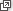## Assumed knowledge

The content of the module Probability, which formalises the ideas in the series of TIMES modules Chance (Year 1–10).

## Motivation

This module introduces discrete random variables. A random variable can be either discrete or continuous. In this module, we cover the first type, and the module Continuous probability distributions covers the second.

The idea of a random variable builds on the fundamental ideas of probability. Students need to understand that random variables are conceptually different from the mathematical variables that they have met before. A random variable is linked to observations in the real world, where uncertainty is involved.

An informal — but important — understanding of a random variable is that it is a variable whose numerical value is determined by the outcome of a random procedure. In this module, we also see the more formal understanding, which is that a random variable is a function from the event space of a random procedure to the set of real numbers.

Random variables are central to the use of probability in practice. They are used to model random phenomena, which means that they are relevant to a wide range of human activity. In particular, they are used extensively in many branches of research, including agriculture, biology, ecology, economics, medicine, meteorology, physics, psychology and others. They provide a structure for making inferences about the world, when it is impossible to measure things comprehensively. They are used to model outcomes of processes that cannot be predicted in advance.

Random variables have distributions. In this module, we describe the essential properties of distributions of discrete random variables. Distributions can have many forms, but there are some special types of distributions that arise in many different practical contexts. In this module, we discuss two of these special distributions: discrete uniform distributions and geometric distributions.

This module also covers the mean of a discrete random variable, which is a measure of central location, and the variance and standard deviation, which are measures of spread.

Next page - Content - Random variables